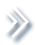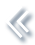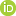####ArchivesReview Articles

## A selective overview of sparse sufficient dimension reduction

Lu Li ,

a East China Normal University, Shanghai, People's Republic of ChinaXuerong Meggie Wen ,

b Missouri University of Science and Technology, Rolla, MO, USAZhou Yu

a East China Normal University, Shanghai, People's Republic of Chinazyu@stat.ecnu.edu.cn

Pages 121-133 | Received 19 Jan. 2020, Accepted 23 Sep. 2020, Published online: 10 Nov. 2020,
• Abstract
• References
• Citations

Abstract

High-dimensional data analysis has been a challenging issue in statistics. Sufficient dimension reduction aims to reduce the dimension of the predictors by replacing the original predictors with a minimal set of their linear combinations without loss of information. However, the estimated linear combinations generally consist of all of the variables, making it difficult to interpret. To circumvent this difficulty, sparse sufficient dimension reduction methods were proposed to conduct model-free variable selection or screening within the framework of sufficient dimension reduction. We review the current literature of sparse sufficient dimension reduction and do some further investigation in this paper.

## References

1. Bondell, H. D., & Li, L. (2009). Shrinkage inverse regression estimation for model–free variable selection. Journal of the Royal Statistical Society: Series B (Statistical Methodology)71(1), 287–299. https://doi.org/10.1111/j.1467-9868.2008.00686.x[Crossref], [Google Scholar]
2. Breiman, L. (1995). Better subset regression using the nonnegative garrote. Technometrics37(4), 373–384. https://doi.org/10.1080/00401706.1995.10484371 [Taylor & Francis Online][Web of Science ®], [Google Scholar]
3. Candes, E., & Tao, T. (2007). The Dantzig selector: Statistical estimation when p is much larger than nThe Annals of Statistics35(6), 2313–2351. https://doi.org/10.1214/009053606000001523 [Crossref][Web of Science ®], [Google Scholar]
4. Chen, J., Stern, M., Wainwright, M. J., & Jordan, M. I. (2017). Kernel feature selection via conditional covariance minimization. In Advances in Neural Information Processing Systems (pp. 6946–6955). [Google Scholar]
5. Chen, X., Zou, C., & Cook, R. D. (2010). Coordinate-independent sparse sufficient dimension reduction and variable selection. The Annals of Statistics38(6), 3696–3723. https://doi.org/10.1214/10-AOS826 [Crossref][Web of Science ®], [Google Scholar]
6. Cook, R. D. (1996). Graphics for regressions with a binary response. Journal of the American Statistical Association91(435), 983–992. https://doi.org/10.1080/01621459.1996.10476968 [Taylor & Francis Online][Web of Science ®], [Google Scholar]
7. Cook, R. D. (1998). Regression graphics. Wiley. [Crossref], [Google Scholar]
8. Cook, R. D. (2004). Testing predictor contributions in sufficient dimension reduction. The Annals of Statistics32(3), 1062–1092. https://doi.org/10.1214/009053604000000292 [Crossref], [Web of Science ®], [Google Scholar]
9. Cook, R. D., & Forzani, L. (2008). Principal fitted components for dimension reduction in regression. Statistical Science23(4), 485–501. https://doi.org/10.1214/08-STS275 [Crossref][Web of Science ®], [Google Scholar]
10. Cook, R. D., & Forzani, L. (2009). Likelihood-based sufficient dimension reduction. Journal of the American Statistical Association104(485), 197–208. https://doi.org/10.1198/jasa.2009.0106 [Taylor & Francis Online][Web of Science ®], [Google Scholar]
11. Cook, R. D., & Ni, L. (2005). Sufficient dimension reduction via inverse regression: A minimum discrepancy approach. Journal of the American Statistical Association100(470), 410–428. https://doi.org/10.1198/016214504000001501 [Taylor & Francis Online][Web of Science ®], [Google Scholar]
12. Cook, R. D., & Weisberg, S. (1991). Sliced inverse regression for dimension reduction: Comment. Journal of the American Statistical Association86(414), 328–332. https://doi.org/10.2307/2290564[Taylor & Francis Online], [Google Scholar]
13. Fan, J., & Li, R. (2001). Variable selection via nonconcave penalized likelihood and its oracle properties. Journal of the American Statistical Association96(456), 1348–1360. https://doi.org/10.1198/016214501753382273 [Taylor & Francis Online][Web of Science ®], [Google Scholar]
14. Fan, J., & Lv, J. (2008). Sure independence screening for ultrahigh dimensional feature space. Journal of the Royal Statistical Society: Series B (Statistical Methodology)70(5), 849–911. https://doi.org/10.1111/rssb.2008.70.issue-5 doi: 10.1111/j.1467-9868.2008.00674.x [Crossref][Web of Science ®], [Google Scholar]
15. Fukumizu, K., Bach, F. R., & Jordan, M. I. (2009). Kernel dimension reduction in regression. The Annals of Statistics37(4), 1871–1905. https://doi.org/10.1214/08-AOS637 [Crossref][Web of Science ®], [Google Scholar]
16. Fukumizu, K., & Leng, C. (2014). Gradient-based kernel dimension reduction for regression. Journal of the American Statistical Association109(505), 359–370. https://doi.org/10.1080/01621459.2013.838167 [Taylor & Francis Online][Web of Science ®], [Google Scholar]
17. Fung, W. K., He, X., Liu, L., & Shi, P. (2002). Dimension reduction based on canonical correlation. Statistica Sinica12(2002), 1093–1113. https://www.jstor.org/stable/24307017. [Google Scholar]
18. Gao, C., Ma, Z., Ren, Z., & Zhou, H. H. (2015). Minimax estimation in sparse canonical correlation analysis. The Annals of Statistics43(5), 2168–2197. https://doi.org/10.1214/15-AOS1332 [Crossref][Web of Science ®], [Google Scholar]
19. Gretton, A., Bousquet, O., Smola, A., & Scholkopf, B. (2005). Measuring statistical dependence with Hilbert-Schmidt norms. In International Conference on Algorithmic Learning Theory (pp. 63–77). Springer. [Google Scholar]
20. Li, K. C. (1991). Sliced inverse regression for dimension reduction. Journal of the American Statistical Association86(414), 316–327. https://doi.org/10.1080/01621459.1991.10475035 [Taylor & Francis Online][Web of Science ®], [Google Scholar]
21. Li, K. C. (1992). On principal Hessian directions for data visualization and dimension reduction: Another application of Stein's lemma. Journal of the American Statistical Association87(420), 1025–1039. https://doi.org/10.1080/01621459.1992.10476258 [Taylor & Francis Online][Web of Science ®], [Google Scholar]
22. Li, K. C. (2000). High dimensional data analysis via the SIR/PHD approach. Lecture Note in Progress. [Google Scholar]
23. Li, L. (2007). Sparse sufficient dimension reduction. Biometrika94(3), 603–613. https://doi.org/10.1093/biomet/asm044 [Crossref][Web of Science ®], [Google Scholar]
24. Li, Z., & Dong, Y. (2020). Model free variable selection with matrix-valued predictors. Journal of Computational and Graphical Statistics27, 1–11. https://doi.org/10.1080/10618600.2020.1806854 [Google Scholar]
25. Li, L., & Nachtsheim, C. J. (2006). Sparse sliced inverse regression. Technometrics48(4), 503–510. https://doi.org/10.1198/004017006000000129 [Taylor & Francis Online][Web of Science ®], [Google Scholar]
26. Li, B., & Wang, S. (2007). On directional regression for dimension reduction. Journal of the American Statistical Association102(479), 997–1008. https://doi.org/10.1198/016214507000000536 [Taylor & Francis Online][Web of Science ®], [Google Scholar]
27. Li, L., & Yin, X. (2008). Sliced inverse regression with regularizations. Biometrics64(1), 124–131. https://doi.org/10.1111/j.1541-0420.2007.00836.x [Crossref][Web of Science ®], [Google Scholar]
28. Li, R., Zhong, W., & Zhu, L. (2012). Feature screening via distance correlation learning. Journal of the American Statistical Association107(499), 1129–1139. https://doi.org/10.1080/01621459.2012.695654 [Taylor & Francis Online][Web of Science ®], [Google Scholar]
29. Lin, Q., Li, X., Huang, D., & Liu, J. S. (2017). On the optimality of sliced inverse regression in high dimensions. arXiv preprint arXiv:1701.06009. [Google Scholar]
30. Lin, Q., Zhao, Z., & Liu, J. (2019). Sparse sliced inverse regression via Lasso. Journal of the American Statistical Association114(528), 1726–1739. https://doi.org/10.1080/01621459.2018.1520115 [Taylor & Francis Online][Web of Science ®], [Google Scholar]
31. Lin, Q., Zhao, Z., & Liu, J. S. (2018). On consistency and sparsity for sliced inverse regression in high dimensions. The Annals of Statistics46(2), 580–610. https://doi.org/10.1214/17-AOS1561 [Crossref][Web of Science ®], [Google Scholar]
32. Ma, Y., & Zhu, L. (2012). A semiparametric approach to dimension reduction. Journal of the American Statistical Association107(497), 168–179. https://doi.org/10.1080/01621459.2011.646925 [Taylor & Francis Online][Web of Science ®], [Google Scholar]
33. Ma, Y., & Zhu, L. (2013a). A review on dimension reduction. International Statistical Review81(1), 134–150. https://doi.org/10.1111/insr.2013.81.issue-1 doi: 10.1111/j.1751-5823.2012.00182.x [Crossref][Web of Science ®], [Google Scholar]
34. Ma, Y., & Zhu, L. (2013b). Efficient estimation in sufficient dimension reduction. The Annals of Statistics41(1), 250–268. https://doi.org/10.1214/12-AOS1072 [Crossref][Web of Science ®], [Google Scholar]
35. Ma, Y., & Zhu, L. (2014). On estimation efficiency of the central mean subspace. Journal of the Royal Statistical Society: Series B (Statistical Methodology)76(5), 885–901. https://doi.org/10.1111/rssb.2014.76.issue-5 doi: 10.1111/rssb.12044 [Crossref][Web of Science ®], [Google Scholar]
36. Ni, L., Cook, R. D., & Tsai, C. L. (2005). A note on shrinkage sliced inverse regression. Biometrika92(1), 242–247. https://doi.org/10.1093/biomet/92.1.242 [Crossref][Web of Science ®], [Google Scholar]
37. Qian, W., Ding, S., & Cook, R. D. (2019). Sparse minimum discrepancy approach to sufficient dimension reduction with simultaneous variable selection in ultrahigh dimension. Journal of the American Statistical Association114(527), 1277–1290. https://doi.org/10.1080/01621459.2018.1497498 [Taylor & Francis Online][Web of Science ®], [Google Scholar]
38. Tan, K., Shi, L., & Yu, Z. (2020). Sparse SIR: Optimal rates and adaptive estimation. The Annals of Statistics48(1), 64–85. https://doi.org/10.1214/18-AOS1791 [Crossref][Web of Science ®], [Google Scholar]
39. Tan, K. M., Wang, Z., Zhang, T., Liu, H., & Cook, R. D. (2018). A convex formulation for high-dimensional sparse sliced inverse regression. Biometrika105(4), 769–782. https://doi-org.ezproxy.uky.edu/10.1093/biomet/asy049[Web of Science ®], [Google Scholar]
40. Tibshirani, R. (1996). Regression shrinkage and selection via the lasso. Journal of the Royal Statistical Society: Series B (Methodological)58(1), 267–288. https://doi.org/10.1111/j.2517-6161.1996.tb02080.x[Crossref][Web of Science ®], [Google Scholar]
41. Wang, H., & Xia, Y. (2008). Sliced regression for dimension reduction. Journal of the American Statistical Association103(482), 811–821. https://doi.org/10.1198/016214508000000418 [Taylor & Francis Online][Web of Science ®], [Google Scholar]
42. Wu, Y., & Li, L. (2011). Asymptotic properties of sufficient dimension reduction with a diverging number of predictors. Statistica Sinica21(2), 707. https://doi.org/10.5705/ss.2011.v21n2a doi: 10.5705/ss.2011.031a [Crossref][Web of Science ®], [Google Scholar]
43. Xia, Y., Tong, H., Li, W. K., & Zhu, L. X. (2002). An adaptive estimation of dimension reduction space. Journal of the Royal Statistical Society: Series B (Statistical Methodology)64(3), 363–410. https://doi.org/10.1111/rssb.2002.64.issue-3 doi: 10.1111/1467-9868.03411 [Crossref][Web of Science ®], [Google Scholar]
44. Yin, X., & Cook, R. D. (2002). Dimension reduction for the conditional kth moment in regression. Journal of the Royal Statistical Society: Series B (Statistical Methodology)64(2), 159–175. https://doi.org/10.1111/rssb.2002.64.issue-2 doi: 10.1111/1467-9868.00330 [Crossref], [Google Scholar]
45. Yin, X., & Cook, R. D. (2003). Estimating central subspaces via inverse third moments. Biometrika90(1), 113–125. https://doi.org/10.1093/biomet/90.1.113 [Crossref][Web of Science ®], [Google Scholar]
46. Yin, X., & Hilafu, H. (2015). Sequential sufficient dimension reduction for large p, small n problems. Journal of the Royal Statistical Society: Series B (Statistical Methodology)77(4), 879–892. https://doi.org/10.1111/rssb.2015.77.issue-4 doi: 10.1111/rssb.12093 [Crossref][Web of Science ®], [Google Scholar]
47. Yin, X., Li, B., & Cook, R. D. (2008). Successive direction extraction for estimating the central subspace in a multiple-index regression. Journal of Multivariate Analysis99(8), 1733–1757. https://doi.org/10.1016/j.jmva.2008.01.006 [Crossref][Web of Science ®], [Google Scholar]
48. Yu, Z., Dong, Y., & Shao, J. (2016). On marginal sliced inverse regression for ultrahigh dimensional model-free feature selection. The Annals of Statistics44(6), 2594–2623. https://doi.org/10.1214/15-AOS1424 [Crossref][Web of Science ®], [Google Scholar]
49. Yu, Z., Dong, Y., & Zhu, L. X. (2016). Trace pursuit: A general framework for model-free variable selection. Journal of the American Statistical Association111(514), 813–821. https://doi.org/10.1080/01621459.2015.1050494 [Taylor & Francis Online][Web of Science ®], [Google Scholar]
50. Yu, Z., Zhu, L., Peng, H., & Zhu, L. (2013). Dimension reduction and predictor selection in semiparametric models. Biometrika100(3), 641–654. https://doi.org/10.1093/biomet/ast005 [Crossref][Web of Science ®], [Google Scholar]
51. Yuan, M., & Lin, Y. (2006). Model selection and estimation in regression with grouped variables. Journal of the Royal Statistical Society: Series B (Statistical Methodology)68(1), 49–67. https://doi.org/10.1111/rssb.2006.68.issue-1 doi: 10.1111/j.1467-9868.2005.00532.x [Crossref][Web of Science ®], [Google Scholar]
52. Zhang, C. H. (2010). Nearly unbiased variable selection under minimax concave penalty. The Annals of Statistics38(2), 894–942. https://doi.org/10.1214/09-AOS729 [Crossref][Web of Science ®], [Google Scholar]
53. Zhou, J., & He, X. (2008). Dimension reduction based on constrained canonical correlation and variable filtering. The Annals of Statistics36(4), 1649–1668. https://doi.org/10.1214/07-AOS529 [Crossref][Web of Science ®], [Google Scholar]
54. Zhu, L. P., Li, L., Li, R., & Zhu, L. X. (2011). Model-free feature screening for ultrahigh-dimensional data. Journal of the American Statistical Association106(496), 1464–1475. https://doi.org/10.1198/jasa.2011.tm10563 [Taylor & Francis Online][Web of Science ®], [Google Scholar]
55. Zhu, L., Miao, B., & Peng, H. (2006). On sliced inverse regression with high-dimensional covariates. Journal of the American Statistical Association101(474), 630–643. https://doi.org/10.1198/016214505000001285 [Taylor & Francis Online][Web of Science ®], [Google Scholar]
56. Zhu, L. P., & Zhu, L. X. (2009a). On distribution–weighted partial least squares with diverging number of highly correlated predictors. Journal of the Royal Statistical Society: Series B (Statistical Methodology)71(2), 525–548. https://doi.org/10.1111/rssb.2009.71.issue-2 doi: 10.1111/j.1467-9868.2008.00697.x [Crossref], [Google Scholar]
57. Zhu, L. P., & Zhu, L. X. (2009b). Nonconcave penalized inverse regression in single-index models with high dimensional predictors. Journal of Multivariate Analysis100(5), 862–875. https://doi.org/10.1016/j.jmva.2008.09.003 [Crossref][Web of Science ®], [Google Scholar]
58. Zou, H. (2006). The adaptive lasso and its oracle properties. Journal of the American Statistical Association101(476), 1418–1429. https://doi.org/10.1198/016214506000000735 [Taylor & Francis Online][Web of Science ®], [Google Scholar]